Explore Fractions Online!return to the Idea Bank index

 NLVM's Fractions Equivalent AppletThe computer selects a fraction and asks you to find an equivalent fraction as you use controls to place another layer of fractional pieces on top of the first fraction.http://nlvm.usu.edu/en/nav/frames_asid_105_g_2_t_1.html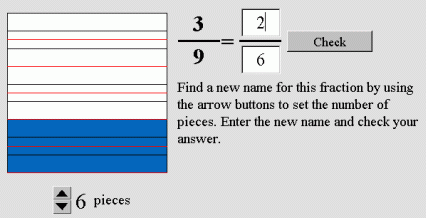Math Playground's Fraction BarsCompare up to four fractions of your choice by splitting each bar into parts and selecting the number of parts to color. http://mathplayground.com/Fraction_bars.html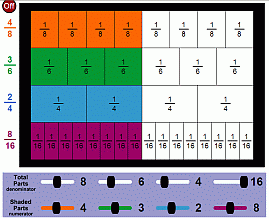Illuminations' Equivalent Fractions
Find two equivalent fractions for a given fraction by dividing two other shapes into parts and coloring parts while arrows point to their values on a number line.
http://illuminations.nctm.org/ActivityDetail.aspx?ID=80

Fraction Bars Applet (Virtual Fractions)
This applet lets you compare up to five fractions, decimals, and/or percents, but it's a bit clumsy to set up.
http://www.arcytech.org/java/fractions/fractions.html

lesson to accompany the Virtual Fractions Applet
http://www.frontiernet.net/~mmankus/talks/bars/fbar1.htm

 Math Playground's Equivalent FractionsFind a fraction between two given fractions by using virtual fraction bars to visualize equivalent fractions with a common denominator. http://mathplayground.com/visual_fractions.html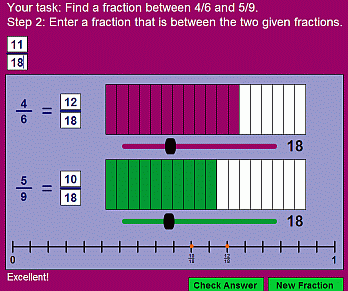Illuminations' Fraction GameTry to move your markers across fraction tracks using the fewest number of fraction cards. http://illuminations.nctm.org/ActivityDetail.aspx?ID=18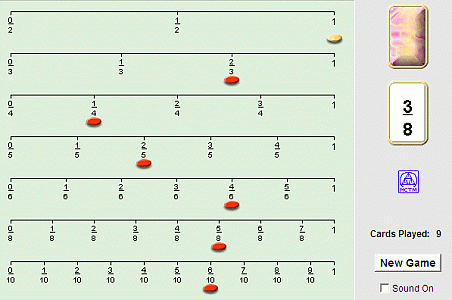NCTM Fraction Model Applet(representing fractions, decimals, and percents): http://my.nctm.org/eresources/repository/2071/applet/FractionPie/index.html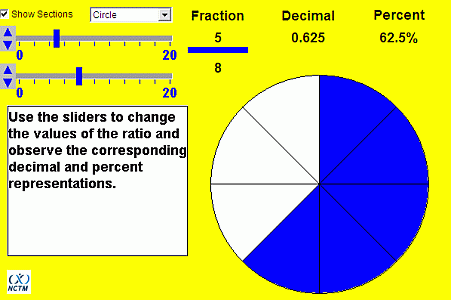Fractional Hi-LoPractice comparing fractions as you try to guess the mystery fraction and find out if each guess is too high or low. http://www.theproblemsite.com/games/hilo.asp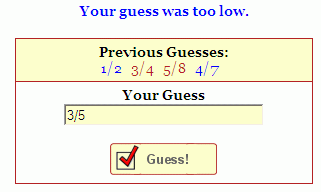Web Math:

Old Egyptian Math Cats Fractions
Solve fraction puzzles the way the Egyptians did - using only numerators of 1.
http://www.mathcats.com/explore/oldegyptianfractions.html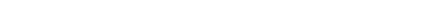top